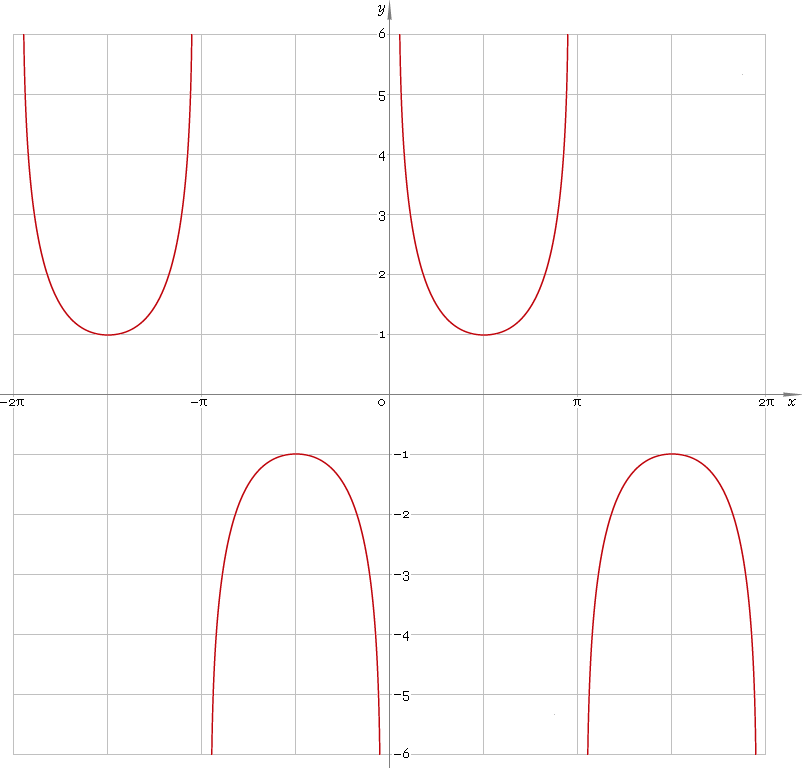The Art of Interface

# csc or cosec — trigonometric cosecant function

Category. Mathematics.

Abstract. Trigonometric cosecant: definition, plot, properties, identities and table of values for some angles.

## 1. Definition

Cosecant of the angle is ratio of the hypotenuse to the opposite leg.

## 2. Plot

Cosecant is 2π periodic function defined everywhere on real axis, except its singular points πn, n=0, ±1, ±2, ... — so, function domain is (πn, π(n + 1)), n∈N. Its stalactite-stalagmite plot is depicted below — fig. 1.Fig. 1. Plot of the cosecant function y = cscx.

Function codomain is entire real axis with gap in the middle: (−∞, −1]∪[1, +∞).

## 3. Identities

Base:

csc−2φ + sec−2φ = 1

and its consequences:

cscφ = ±1 /√(1 − cos2φ)
cscφ = ±√(1 + tan2φ) / tanφ
cscφ = ±√(1 + cot2φ)
cscφ = ±secφ /√(sec2φ − 1)

By definition:

cscφ ≡ 1 /sinφ

Properties — symmetry, periodicity, etc.:

csc−φ = −cscφ
cscφ = csc(φ + 2πn), where n = 0, ±1, ±2, ...
cscφ = csc(π − φ)
cscφ = −csc(π + φ)
cscφ = sec(π/2 − φ)

Sum of angles:

csc(φ + ψ + χ) = secφ secψ secχ / (tanφ + tanψ + tanχ − tanφ tanψ tanχ)

Some angles:

Angle φValue cscφ
π/12√6 + √2
π/10√5 + 1
π/8√(4 + 2√2)
π/62
π/5√(50 + 10√5) /5
π/4√2
3π/10√5 − 1
π/32√3 /3
3π/8√(2 − √2)
2π/5√(50 − 10√5) /5
5π/12√6 − √2
π/21
Table 1. Cosecant for some angles.

## 4. Support

Trigonometric cosecant function csc or cosec of the real argument is supported by free version of the Librow calculator.

Trigonometric cosecant function csc or cosec of the complex argument is supported by professional version of the Librow calculator.

## 5. How to use

To calculate cosecant of the number:

``csc(-1);``

To calculate cosecant of the current result:

``csc(rslt);``

To calculate cosecant of the angle φ in memory:

``csc(mem[φ]);``